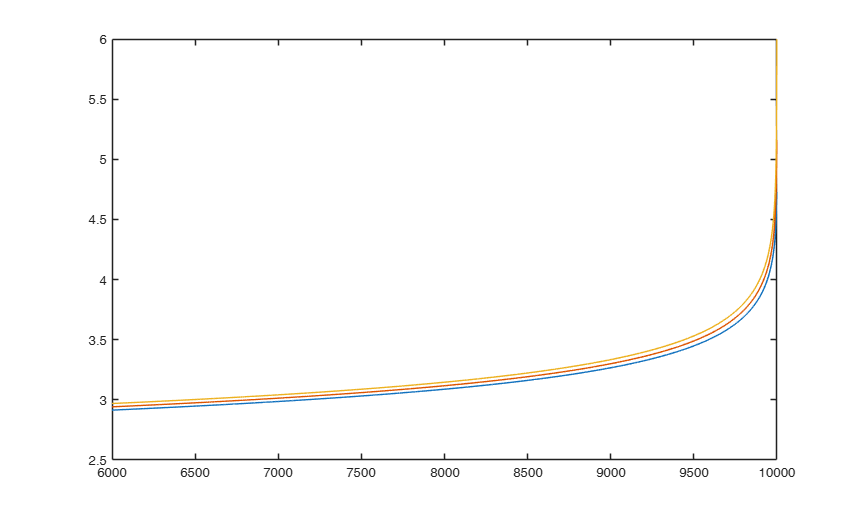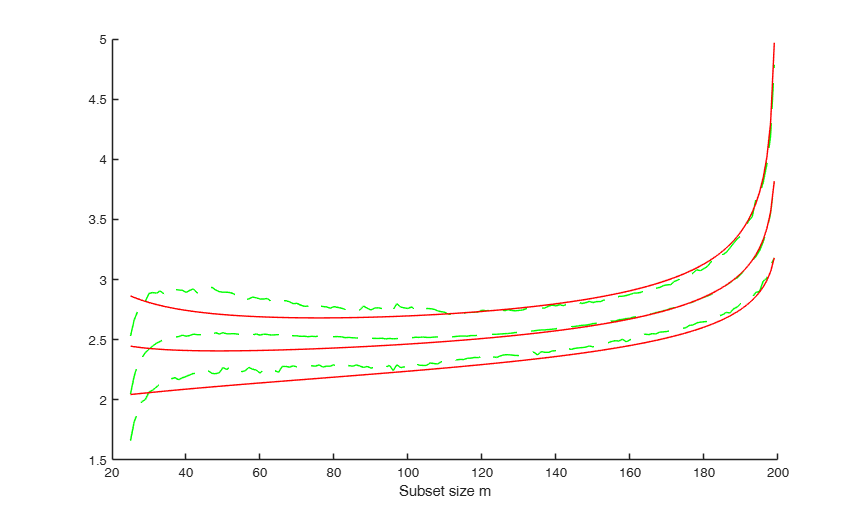# FSMenvmmd

FSMenvmmd computes the theoretical envelopes of Minimum MD outside subset during the search

## Syntax

• MMDenv=FSMenvmmd(n,v)example
• MMDenv=FSMenvmmd(n,v,Name,Value)example

## Description

 MMDenv =FSMenvmmd(n, v) FSMenvmmd with all default options.

 MMDenv =FSMenvmmd(n, v, Name, Value) FSMenvmmd with otpional arguments.

## Examples

expand all

###FSMenvmmd with all default options.

Example of creation of 1 per cent, 50 per cent and 99 per cent envelopes based on 10000 observations and 5 explanatory variables.

MMDenv=FSMenvmmd(10000,5);
plot(MMDenv(:,1),MMDenv(:,2:end))###FSMenvmmd with otpional arguments.

Example of creation of 1 per cent, 50 per cent and 99 per cent envelopes based on 10000 observations and 5 explanatory variables. The envelopes are produced for scaled Mahalanobis distances (no consistency factor is applied)

MMDenv=FSMenvmmd(10000,5,'scaled',1);
plot(MMDenv(:,1),MMDenv(:,2:end))## Related Examples

expand all

###Order statistics and simulations envelopes .

In this example we compare the accuracy of the envelopes computed with order statistics with those which come from simulations.

% Fix a seed
state=1000;
mtstream = RandStream('shr3cong','Seed',state);
RandStream.setGlobalStream(mtstream);
defaultStream = RandStream.getGlobalStream();
reset(defaultStream)
% If you run this example in a version older than 7.9 replace the previous
% four lines with
% randn('state', 1000);
n=200;
p=3;
init=25;
nsimul=1000;
mmdStore=zeros(n-init,nsimul);
for j=1:nsimul
Y=randn(n,p);
[fre]=unibiv(Y);
%create an initial subset with the 20 observations with the lowest
%Mahalanobis Distance
fre=sortrows(fre,4);
bs=fre(1:25,1);
mmd = FSMmmd(Y,bs,'init',init);
mmdStore(:,j)=mmd(:,2);
end
% Sort rows of matrix mmdStore
mmdStore=sort(mmdStore,2);
% Create figure which compares empirical and theoretical forward envelopes
% for minimum deletion residual
figure;
hold('on');
quant=[0.01 0.5 0.99];
sel=round(nsimul*quant);
% Plot lines of empirical quantiles
line(mmd(:,1),mmdStore(:,sel),'LineStyle','--','Color','g');
% Plots lines of theoretical quantiles using order statistics
mmdT=FSMenvmmd(n,p,'init',init);
line(mmdT(:,1),mmdT(:,2:4),'LineStyle','-','Color','r');
xlabel('Subset size m');## Input Arguments

### n — Number of observations. Scalar.

Number of observations.

Data Types: single | double

### v — Number of variables. Scalar.

Number of variables.

Data Types: single | double

### Name-Value Pair Arguments

Specify optional comma-separated pairs of Name,Value arguments. Name is the argument name and Value is the corresponding value. Name must appear inside single quotes (' '). You can specify several name and value pair arguments in any order as  Name1,Value1,...,NameN,ValueN.

Example:  'init',50 , 'prob',[0.05 0.95] , 'scaled',false 

### init —Point where to start monitoring required diagnostics.scalar.

Note that if bsb is supplied, init>=length(bsb). If init is not specified it will be set equal to floor(n*0.6).

Example:  'init',50 

Data Types: double

### prob —quantiles for which envelopes have to be computed.vector.

Vector containing 1 x k elements .

The default is to produce 1 per cent, 50 per cent and 99 per cent envelopes.

Example:  'prob',[0.05 0.95] 

Data Types: double

### scaled —It indicates how to compute the envelopes.boolean.

If scaled=true0 the envelopes are produced for scaled Mahalanobis distances (no consistency factor is applied) else the traditional consistency factor is applied (this is the default)

Example:  'scaled',false 

Data Types: logical

## Output Arguments

### MMDenv —Envelopes for the requested quantiles. n-m0+1 x length(prob)+1 columns

1st col = fwd search index from m0 to n-1;

2nd col = envelope for quantile prob;

3rd col = envelope for quantile prob;

...;

(k+1) col = envelope for quantile prob[k].

Riani, M., Atkinson, A.C. and Cerioli, A. (2009), Finding an unknown number of multivariate outliers, "Journal of the Royal Statistical Society Series B", Vol. 71, pp. 201-221.